# NCERT Exemplar Solutions for Class 6 Maths Chapter 5 Data Handling

NCERT Exemplar Solutions for Class 6 Maths Chapter 5 Data Handling, are available in pdf format. students can check for the answers, whenever they are facing difficulty while answering the questions. These Solutions help students to clear doubts quickly and assist in learning the topic effectively. Students of Class 6 are suggested to practice NCERT Exemplar Solutions for Class 6 Maths to strengthen their fundamentals and be able to solve questions that are usually asked in the examination.

Chapter 5 – Data Handling, the data can be arranged in a tabular form using tally marks. In a bar graph, bars of uniform width are drawn horizontally or vertically with equal spacing between them. Length of each bar gives the required information. Important topics covered in this chapter are,

• A pictograph represents data through pictures of objects.
• Interpretation of a pictograph and drawing a pictograph.
• Representation of data using a bar diagram or bar graph.
• Interpretation of a bar graph and drawing a bar graph.

## Download the PDF of NCERT Exemplar Solutions for Class 6 Maths Chapter 5 Data Handling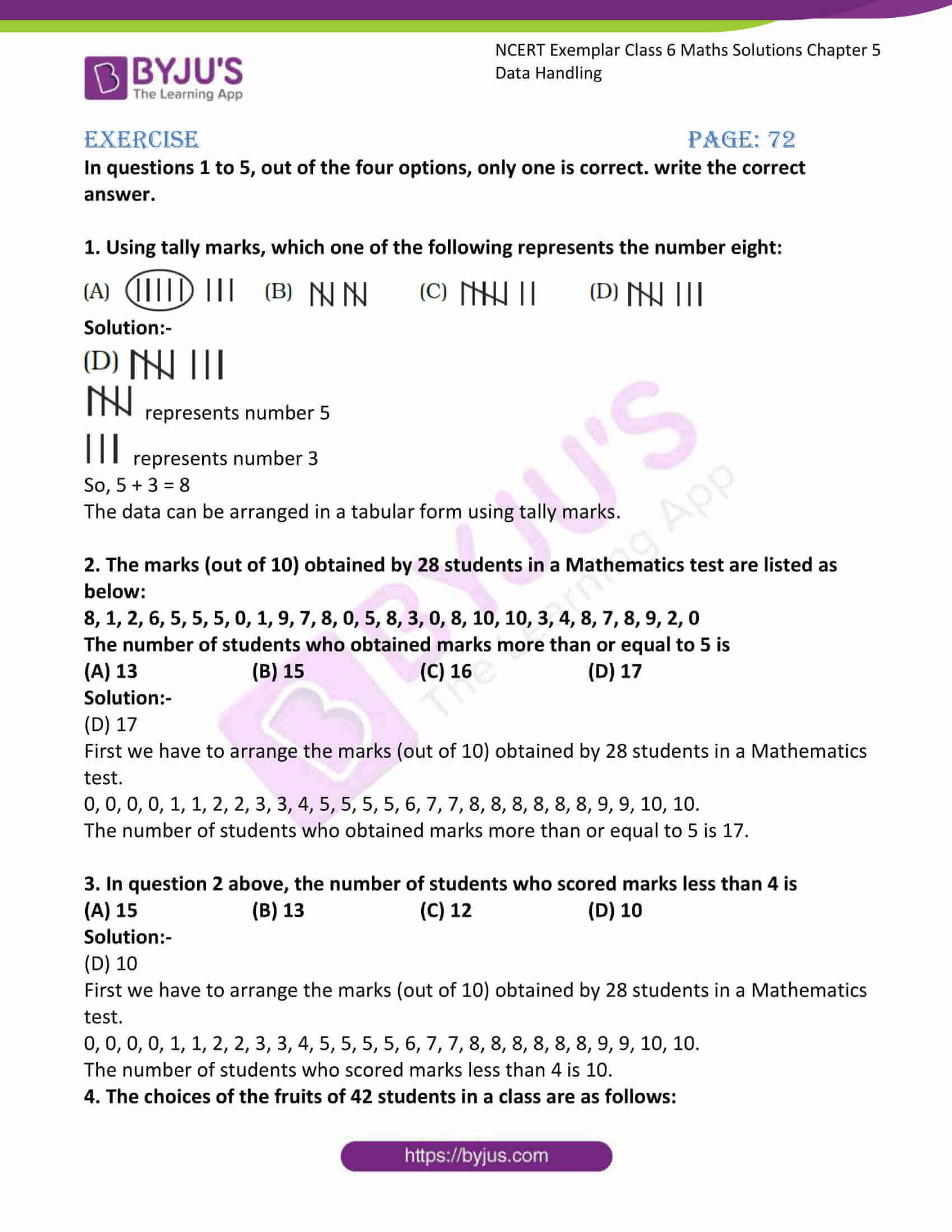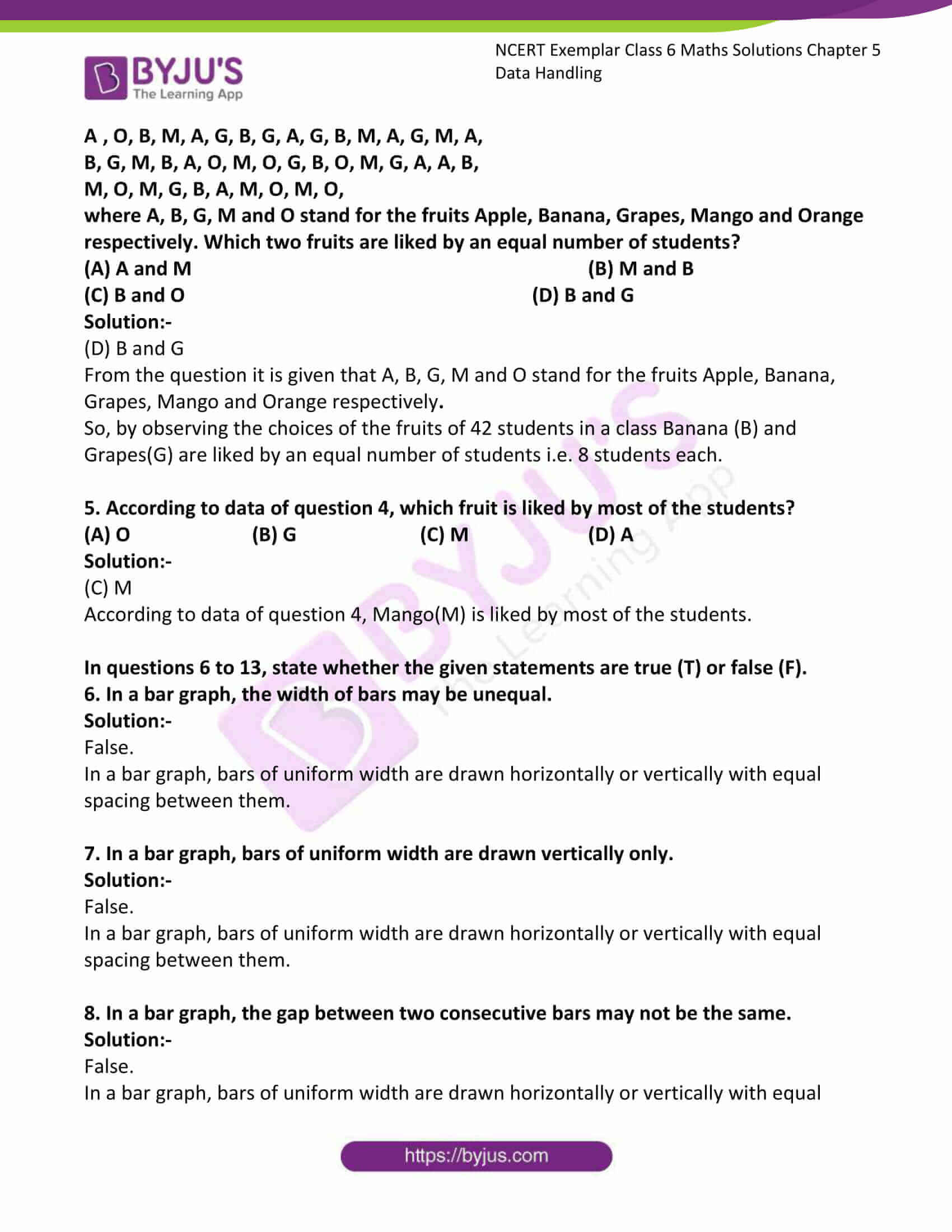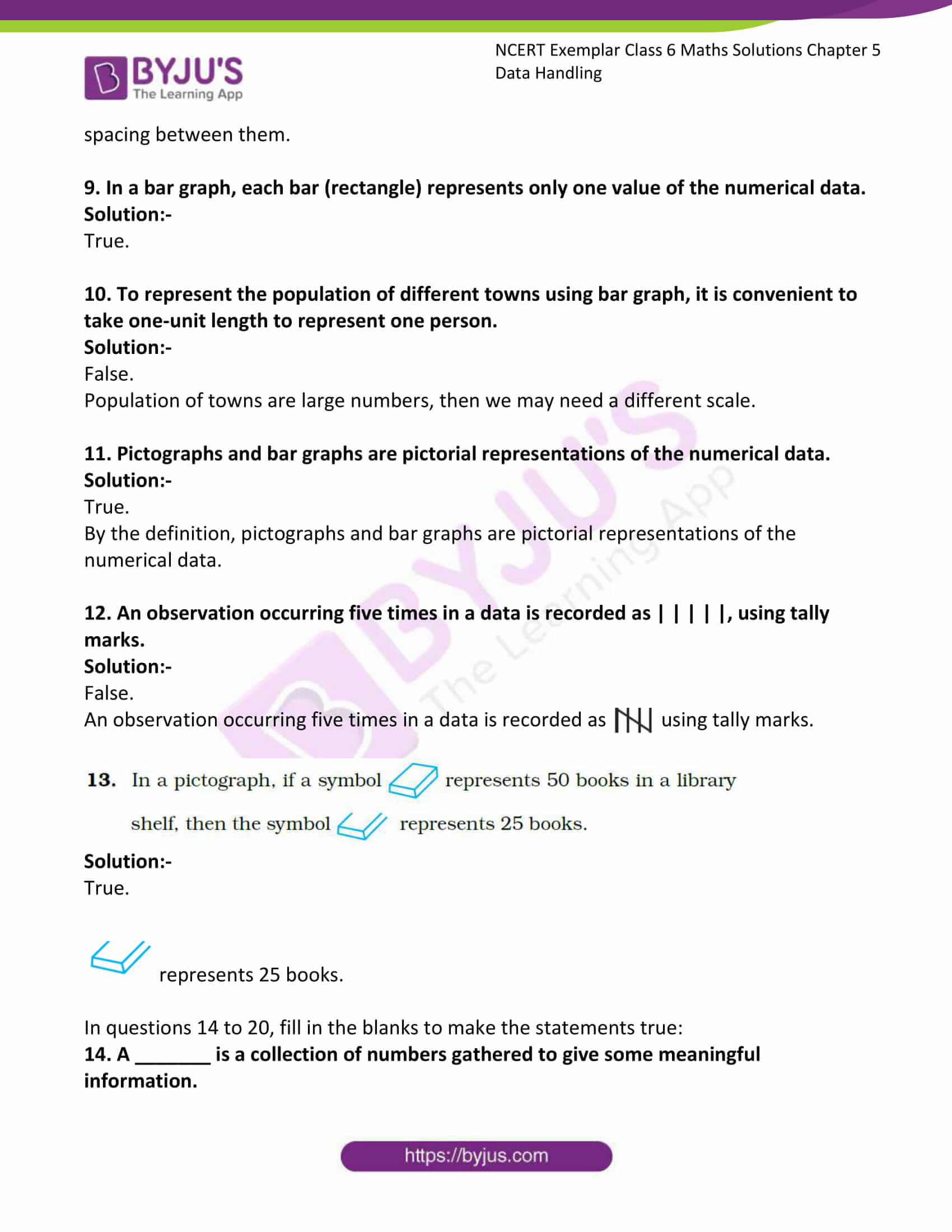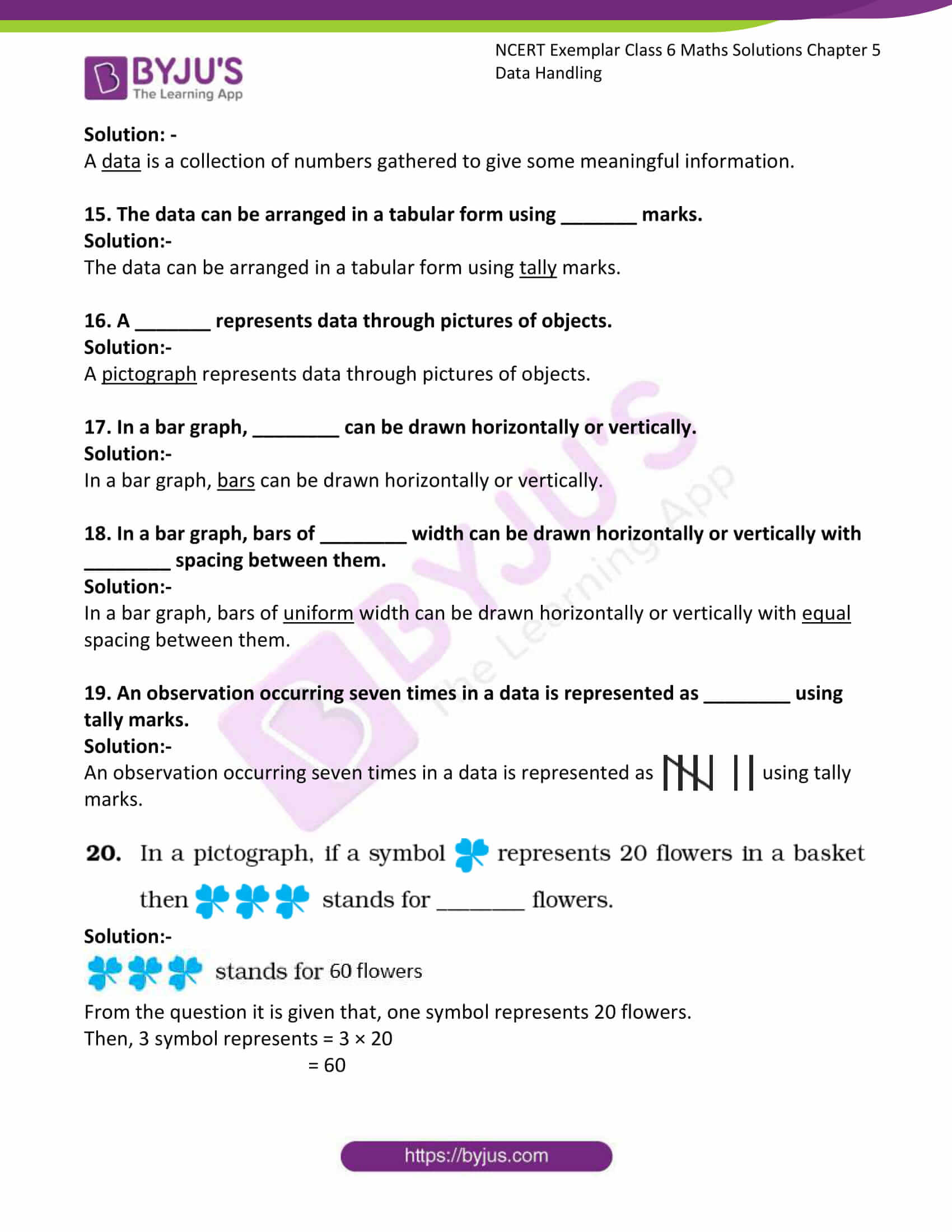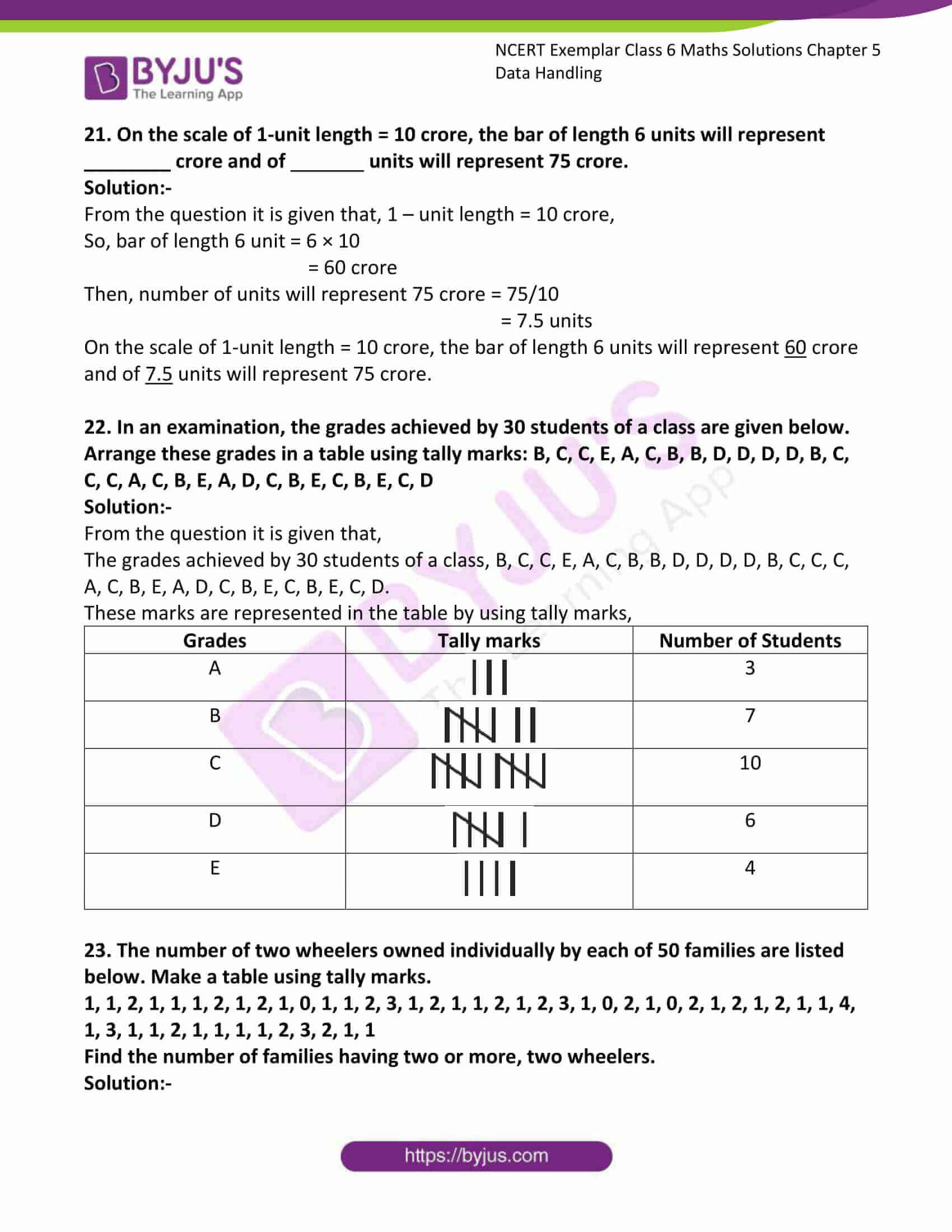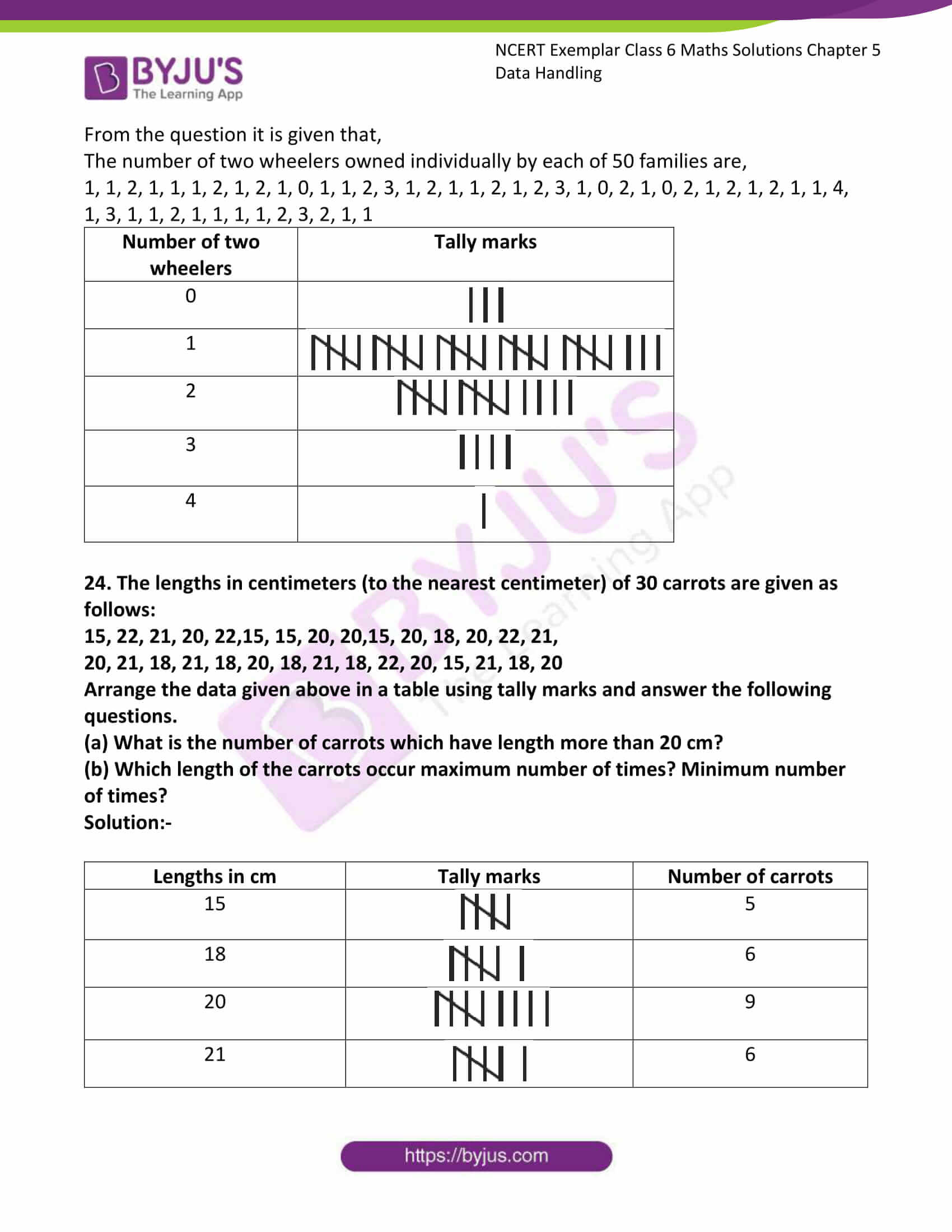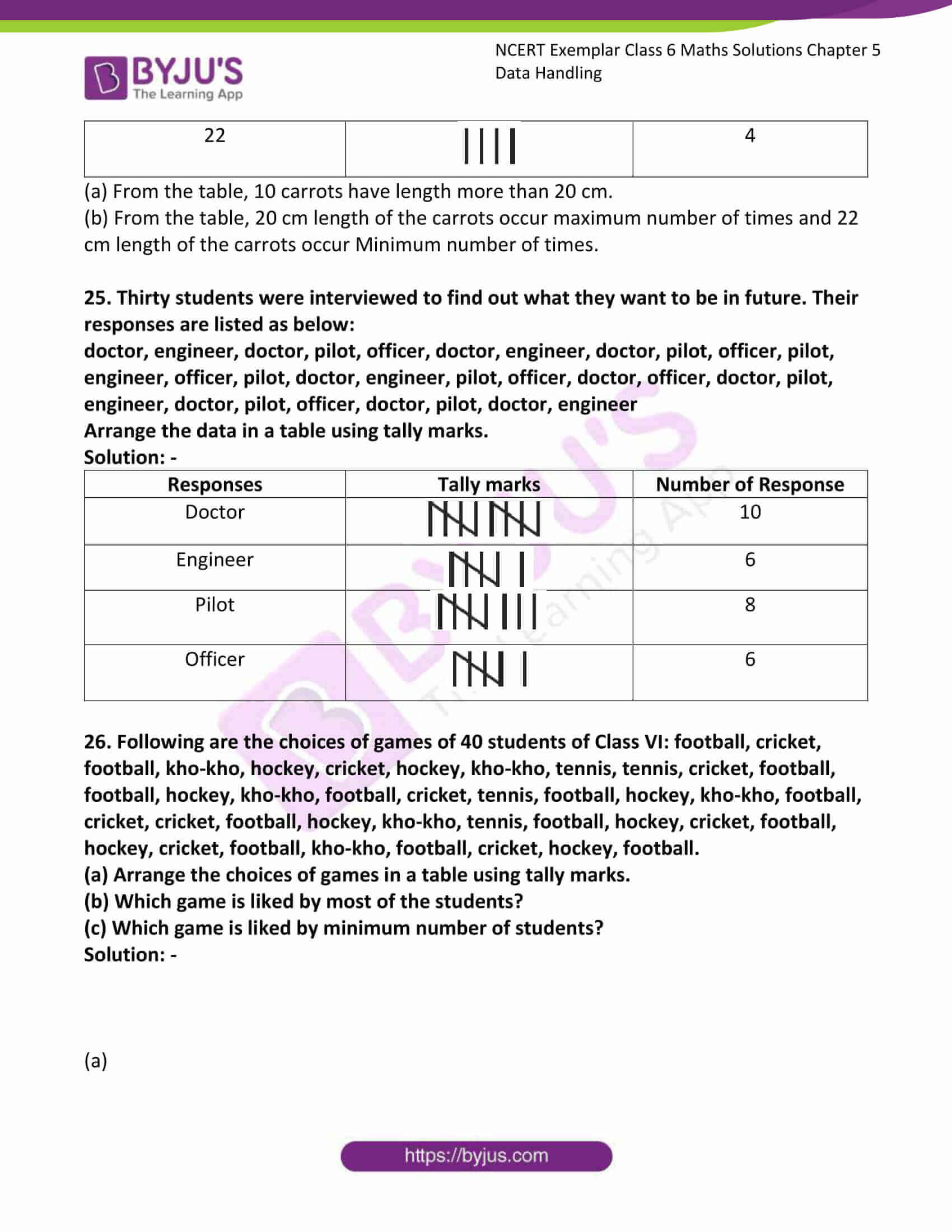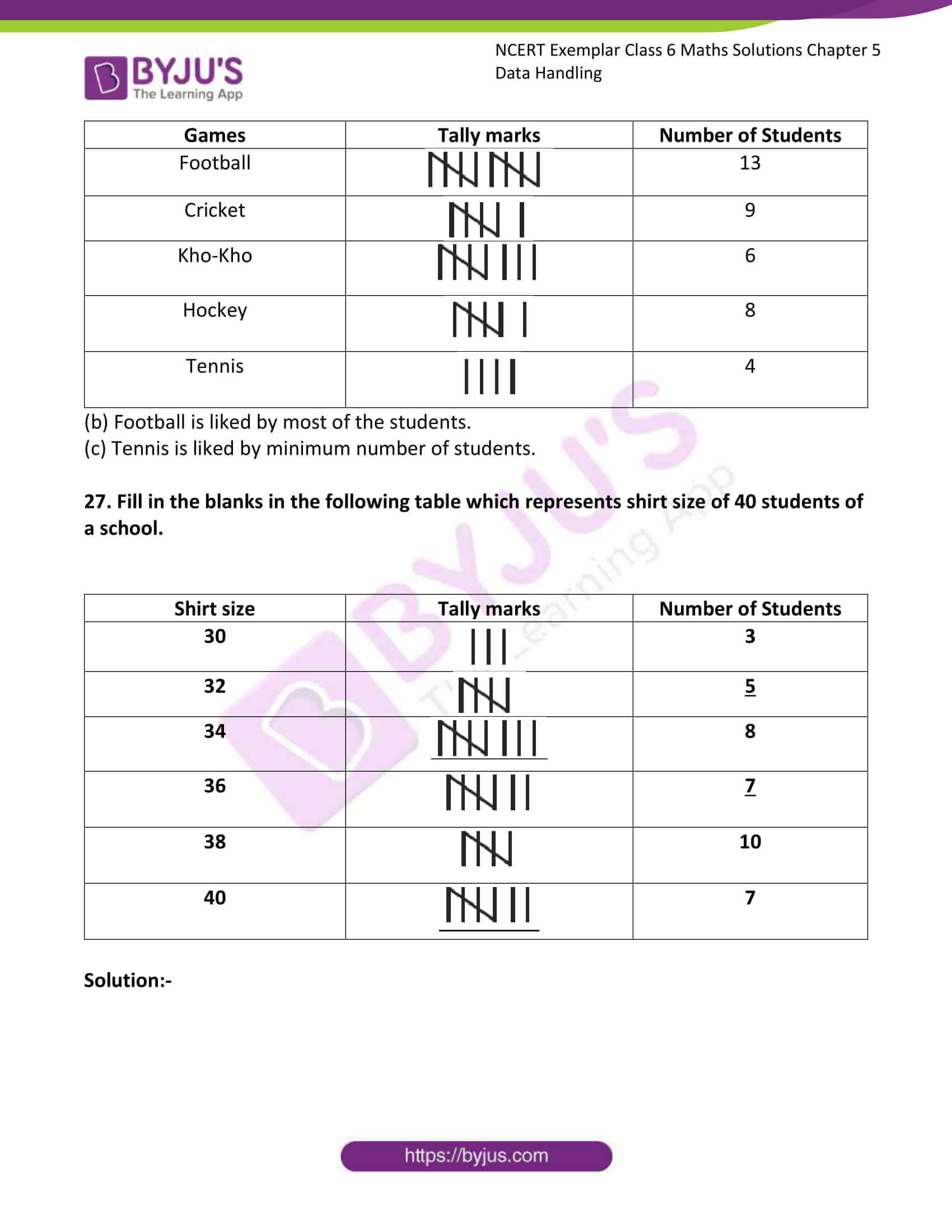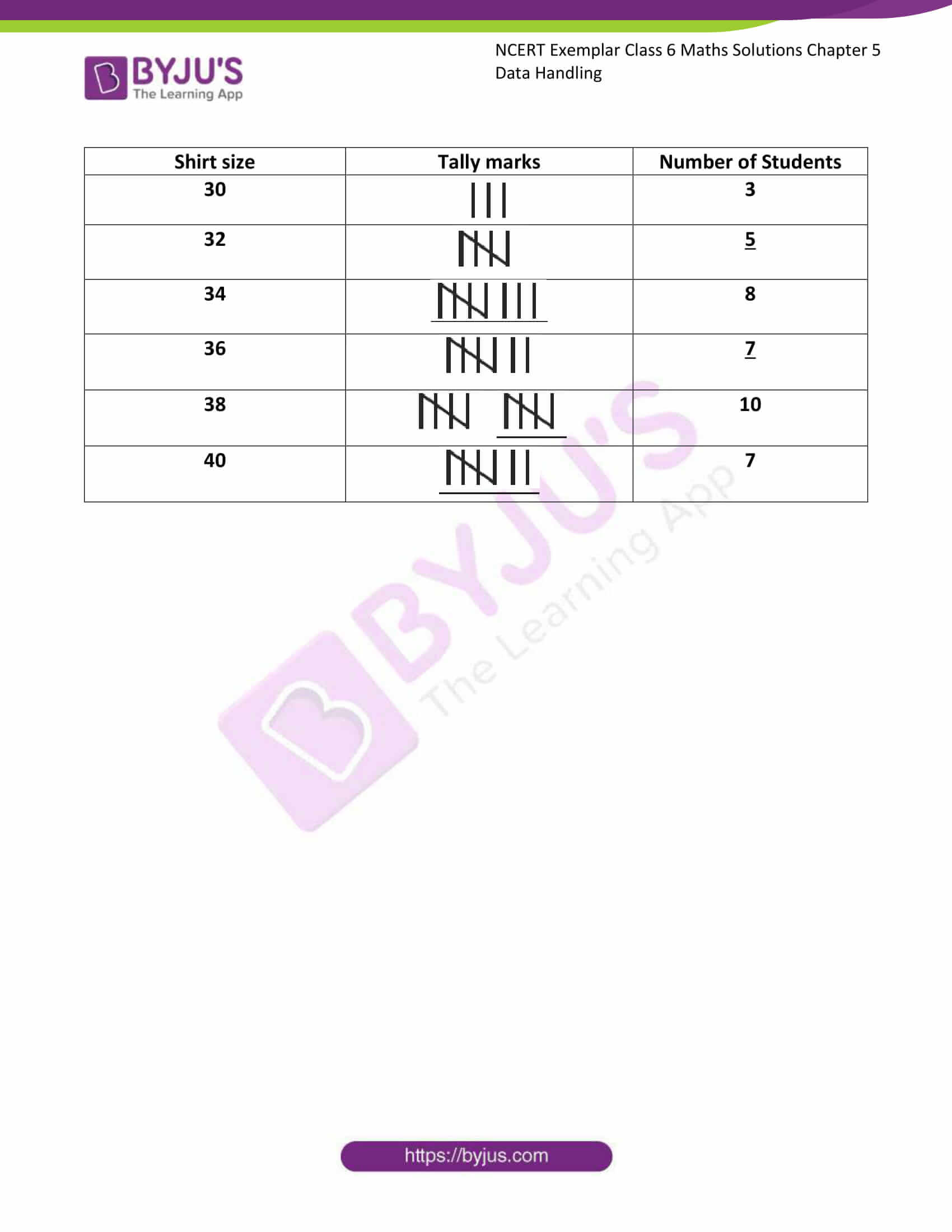### Access answers to Maths NCERT Exemplar Solutions for Class 6 Maths Chapter 5 Data Handling

Exercise Page: 72

In questions 1 to 5, out of the four options, only one is correct. write the correct answer.

1. Using tally marks, which one of the following represents the number eight: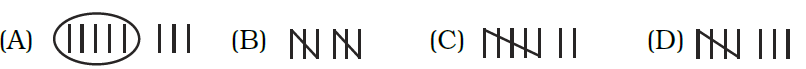Solution:-represents number 5represents number 3

So, 5 + 3 = 8

The data can be arranged in a tabular form using tally marks.

2. The marks (out of 10) obtained by 28 students in a Mathematics test are listed as below:

8, 1, 2, 6, 5, 5, 5, 0, 1, 9, 7, 8, 0, 5, 8, 3, 0, 8, 10, 10, 3, 4, 8, 7, 8, 9, 2, 0

The number of students who obtained marks more than or equal to 5 is

(A) 13 (B) 15 (C) 16 (D) 17

Solution:-

(D) 17

First we have to arrange the marks (out of 10) obtained by 28 students in a Mathematics test.

0, 0, 0, 0, 1, 1, 2, 2, 3, 3, 4, 5, 5, 5, 5, 6, 7, 7, 8, 8, 8, 8, 8, 8, 9, 9, 10, 10.

The number of students who obtained marks more than or equal to 5 is 17.

3. In question 2 above, the number of students who scored marks less than 4 is

(A) 15 (B) 13 (C) 12 (D) 10

Solution:-

(D) 10

First we have to arrange the marks (out of 10) obtained by 28 students in a Mathematics test.

0, 0, 0, 0, 1, 1, 2, 2, 3, 3, 4, 5, 5, 5, 5, 6, 7, 7, 8, 8, 8, 8, 8, 8, 9, 9, 10, 10.

The number of students who scored marks less than 4 is 10.

4. The choices of the fruits of 42 students in a class are as follows:

A, O, B, M, A, G, B, G, A, G, B, M, A, G, M, A,

B, G, M, B, A, O, M, O, G, B, O, M, G, A, A, B,

M, O, M, G, B, A, M, O, M, O,

where A, B, G, M and O stand for the fruits Apple, Banana, Grapes, Mango and Orange respectively. Which two fruits are liked by an equal number of students?

(A) A and M (B) M and B

(C) B and O (D) B and G

Solution:-

(D) B and G

From the question it is given that A, B, G, M and O stand for the fruits Apple, Banana, Grapes, Mango and Orange respectively.

So, by observing the choices of the fruits of 42 students in a class Banana (B) and Grapes(G) are liked by an equal number of students i.e. 8 students each.

5. According to data of question 4, which fruit is liked by most of the students?

(A) O (B) G (C) M (D) A

Solution:-

(C) M

According to data of question 4, Mango(M) is liked by most of the students.

In questions 6 to 13, state whether the given statements are true (T) or false (F).

6. In a bar graph, the width of bars may be unequal.

Solution:-

False.

In a bar graph, bars of uniform width are drawn horizontally or vertically with equal spacing between them.

7. In a bar graph, bars of uniform width are drawn vertically only.

Solution:-

False.

In a bar graph, bars of uniform width are drawn horizontally or vertically with equal spacing between them.

8. In a bar graph, the gap between two consecutive bars may not be the same.

Solution:-

False.

In a bar graph, bars of uniform width are drawn horizontally or vertically with equal spacing between them.

9. In a bar graph, each bar (rectangle) represents only one value of the numerical data.

Solution:-

True.

10. To represent the population of different towns using bar graph, it is convenient to take one-unit length to represent one person.

Solution:-

False.

Population of towns are large numbers, then we may need a different scale.

11. Pictographs and bar graphs are pictorial representations of the numerical data.

Solution:-

True.

By the definition, pictographs and bar graphs are pictorial representations of the numerical data.

12. An observation occurring five times in a data is recorded as | | | | |, using tally marks.

Solution:-

False.

An observation occurring five times in a data is recorded as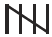using tally marks.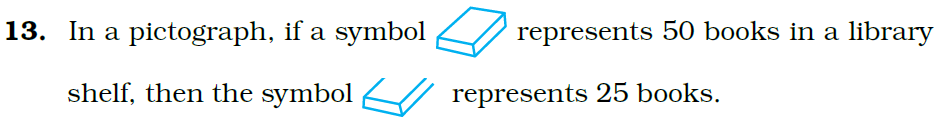Solution:-

True.represents 25 books.

In questions 14 to 20, fill in the blanks to make the statements true:

14. A _______ is a collection of numbers gathered to give some meaningful information.

Solution: –

A data is a collection of numbers gathered to give some meaningful information.

15. The data can be arranged in a tabular form using _______ marks.

Solution:-

The data can be arranged in a tabular form using tally marks.

16. A _______ represents data through pictures of objects.

Solution:-

A pictograph represents data through pictures of objects.

17. In a bar graph, ________ can be drawn horizontally or vertically.

Solution:-

In a bar graph, bars can be drawn horizontally or vertically.

18. In a bar graph, bars of ________ width can be drawn horizontally or vertically with ________ spacing between them.

Solution:-

In a bar graph, bars of uniform width can be drawn horizontally or vertically with equal spacing between them.

19. An observation occurring seven times in a data is represented as ________ using tally marks.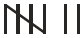Solution:-

An observation occurring seven times in a data is represented as using tally marks.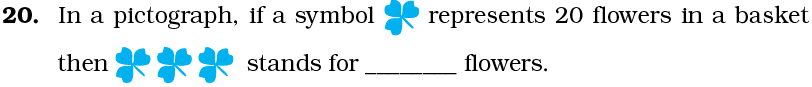Solution:-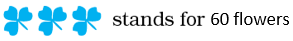From the question it is given that, one symbol represents 20 flowers.

Then, 3 symbol represents = 3 × 20

= 60

21. On the scale of 1-unit length = 10 crore, the bar of length 6 units will represent ________ crore and of units will represent 75 crore.

Solution:-

From the question it is given that, 1 – unit length = 10 crore,

So, bar of length 6 unit = 6 × 10

= 60 crore

Then, number of units will represent 75 crore = 75/10

= 7.5 units

On the scale of 1-unit length = 10 crore, the bar of length 6 units will represent 60 crore and of 7.5 units will represent 75 crore.

22. In an examination, the grades achieved by 30 students of a class are given below. Arrange these grades in a table using tally marks: B, C, C, E, A, C, B, B, D, D, D, D, B, C, C, C, A, C, B, E, A, D, C, B, E, C, B, E, C, D

Solution:-

From the question it is given that,

The grades achieved by 30 students of a class, B, C, C, E, A, C, B, B, D, D, D, D, B, C, C, C, A, C, B, E, A, D, C, B, E, C, B, E, C, D.

These marks are represented in the table by using tally marks,

 Grades Tally marks Number of Students A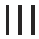3 B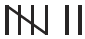7 C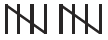10 D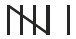6 E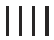4

23. The number of two wheelers owned individually by each of 50 families are listed below. Make a table using tally marks.

1, 1, 2, 1, 1, 1, 2, 1, 2, 1, 0, 1, 1, 2, 3, 1, 2, 1, 1, 2, 1, 2, 3, 1, 0, 2, 1, 0, 2, 1, 2, 1, 2, 1, 1, 4, 1, 3, 1, 1, 2, 1, 1, 1, 1, 2, 3, 2, 1, 1

Find the number of families having two or more, two wheelers.

Solution:-

From the question it is given that,

The number of two wheelers owned individually by each of 50 families are,

1, 1, 2, 1, 1, 1, 2, 1, 2, 1, 0, 1, 1, 2, 3, 1, 2, 1, 1, 2, 1, 2, 3, 1, 0, 2, 1, 0, 2, 1, 2, 1, 2, 1, 1, 4, 1, 3, 1, 1, 2, 1, 1, 1, 1, 2, 3, 2, 1, 1

 Number of two wheelers Tally marks 01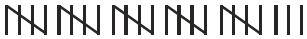2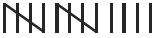3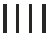424. The lengths in centimeters (to the nearest centimeter) of 30 carrots are given as follows:

15, 22, 21, 20, 22,15, 15, 20, 20,15, 20, 18, 20, 22, 21,

20, 21, 18, 21, 18, 20, 18, 21, 18, 22, 20, 15, 21, 18, 20

Arrange the data given above in a table using tally marks and answer the following questions.

(a) What is the number of carrots which have length more than 20 cm?

(b) Which length of the carrots occur maximum number of times? Minimum number of times?

Solution:-

 Lengths in cm Tally marks Number of carrots 155 186 20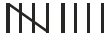9 216 224

(a) From the table, 10 carrots have length more than 20 cm.

(b) From the table, 20 cm length of the carrots occur maximum number of times and 22 cm length of the carrots occur Minimum number of times.

25. Thirty students were interviewed to find out what they want to be in future. Their responses are listed as below:

doctor, engineer, doctor, pilot, officer, doctor, engineer, doctor, pilot, officer, pilot, engineer, officer, pilot, doctor, engineer, pilot, officer, doctor, officer, doctor, pilot, engineer, doctor, pilot, officer, doctor, pilot, doctor, engineer

Arrange the data in a table using tally marks.

Solution: –

 Responses Tally marks Number of Response Doctor10 Engineer6 Pilot8 Officer6

26. Following are the choices of games of 40 students of Class VI: football, cricket, football, kho-kho, hockey, cricket, hockey, kho-kho, tennis, tennis, cricket, football, football, hockey, kho-kho, football, cricket, tennis, football, hockey, kho-kho, football, cricket, cricket, football, hockey, kho-kho, tennis, football, hockey, cricket, football, hockey, cricket, football, kho-kho, football, cricket, hockey, football.

(a) Arrange the choices of games in a table using tally marks.

(b) Which game is liked by most of the students?

(c) Which game is liked by minimum number of students?

Solution: –

(a)

 Games Tally marks Number of Students Football13 Cricket9 Kho-Kho6 Hockey8 Tennis4

(b) Football is liked by most of the students.

(c) Tennis is liked by minimum number of students.

27. Fill in the blanks in the following table which represents shirt size of 40 students of a school.

 Shirt size Tally marks Number of Students 303 325 348 367 3810 407

Solution:-

 Shirt size Tally marks Number of Students 303 325 348 367 3810 407